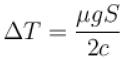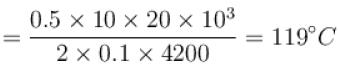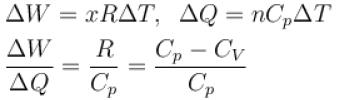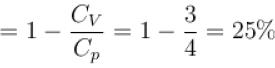Courses

# First Law Of Thermodynamics MCQ Level – 2 (Part - 1)

## 10 Questions MCQ Test Topic wise Tests for IIT JAM Physics | First Law Of Thermodynamics MCQ Level – 2 (Part - 1)

Description
This mock test of First Law Of Thermodynamics MCQ Level – 2 (Part - 1) for Physics helps you for every Physics entrance exam. This contains 10 Multiple Choice Questions for Physics First Law Of Thermodynamics MCQ Level – 2 (Part - 1) (mcq) to study with solutions a complete question bank. The solved questions answers in this First Law Of Thermodynamics MCQ Level – 2 (Part - 1) quiz give you a good mix of easy questions and tough questions. Physics students definitely take this First Law Of Thermodynamics MCQ Level – 2 (Part - 1) exercise for a better result in the exam. You can find other First Law Of Thermodynamics MCQ Level – 2 (Part - 1) extra questions, long questions & short questions for Physics on EduRev as well by searching above.
QUESTION: 1

### One mole of an ideal gas expands adiabatically from temperature T1 to temperature T2. The work done by the gas is Select one:

Solution: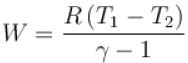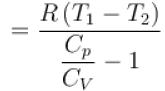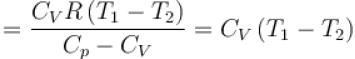The correct answer is: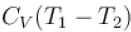QUESTION: 2

### The pressure-volume graph of an ideal gas cycle consisting of isothermal and adiabatic process is shown in the figure. The adiabatic process is described by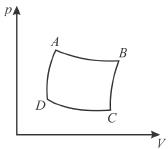Select one:

Solution:

BC and DA represent the adiabatic expansion and the compression since we know that the adiabatic curves are more steeper than isothermal curves.

QUESTION: 3

### A water fall is 168m high. Assuming that half the kinetic energy of the falling water gets converted into heat, the rise in temperature of water is approximately (take g = 10m/s2) Select one:

Solution: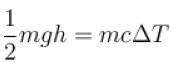⇒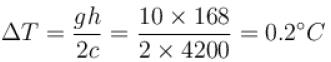QUESTION: 4

One gram of water on evaporation at atmospheric pressure forms 1671cm3 of steam. Heat of vaporization at this pressure is 540 cal/g. Calculate the increase in the internal energy. (Atmospheric pressure = 1×105N/m2)

Select one:

Solution: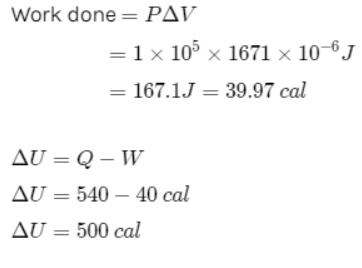QUESTION: 5

A sample of gas expands from volume V1 to V2. The amount of work done by the gas in greatest when the expansion is.
Select one:

Solution:

pV curves for 3 processes are show is below. Note that the area under isobaric curve is maximum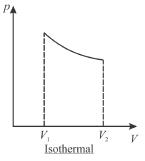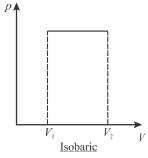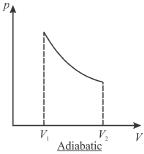QUESTION: 6

One gram of water on evaporation at atmospheric pressure forms 1671cm3 of steam. Heat of vaporization at this pressure is 540 cal/g. The increase in internal energy is
Select one:

Solution: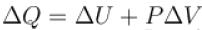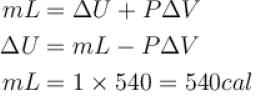or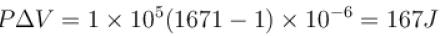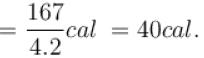∴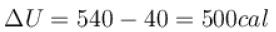The correct answer is: 500 cal

QUESTION: 7

Heat capacity of a substance is infinite. It means :
Select one:

Solution:
QUESTION: 8

A system changes from the state (p1, V1 ) to the state (p2, V2 ) as shown in the figure. The work done by the system is.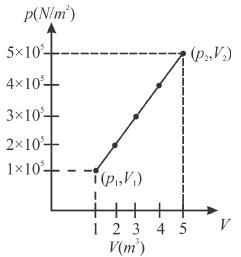Select one:

Solution: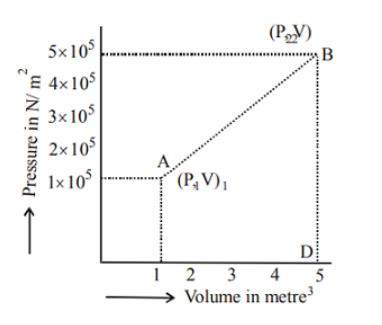Work done = Area of graph ABD;
From above we get,
Area = 1/2 × 4 (1 × 105 + 5 × 105) = 2 × 6 × 105   = 12 × 105J

QUESTION: 9

A body of mass 25kg is dragged on a rough horizontal road for one hour with a speed of 20km/hr.
If the coefficient of friction is 0.5 and half of the heat produced is absorbed by the body, the rise in its temperature is (specific heat of body = 0.1cal/g ºC, g = 10m/s2)
Select one:

Solution:

Distance traveled in one hour,
S = 20km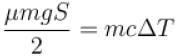Now,⇒